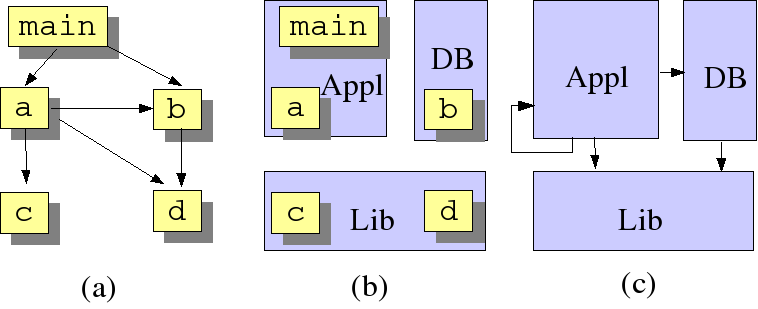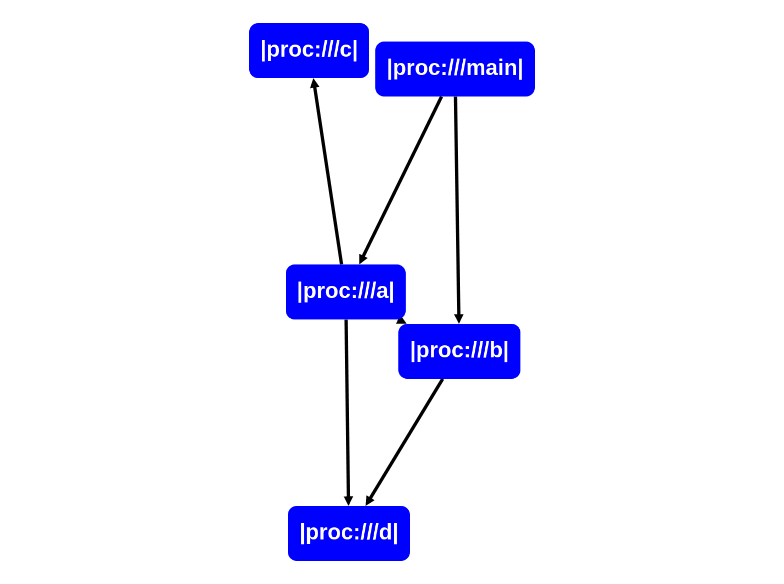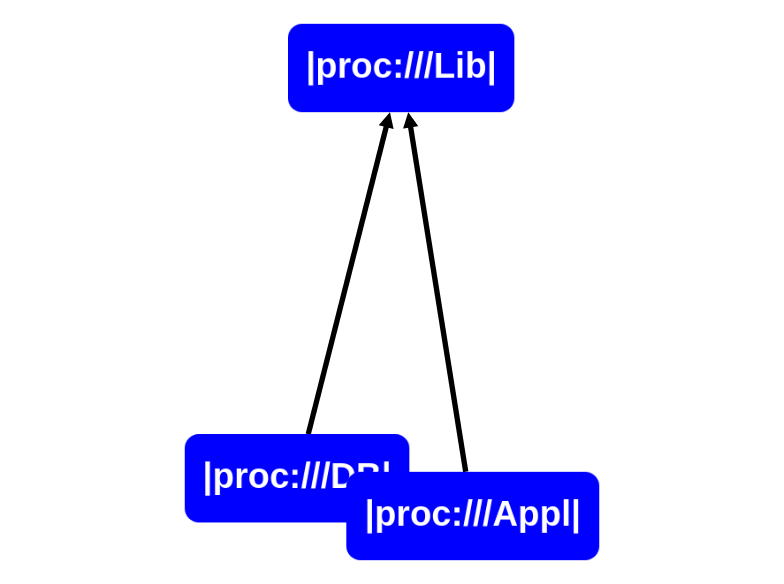# Call Lifting

rascal-0.33.7

#### Synopsis​

Lift procedure calls to component calls.

#### Description​

A frequently occurring problem is that we know the call relation of a system but that we want to understand it at the component level rather than at the procedure level. If it is known to which component each procedure belongs, it is possible to lift the call relation to the component level. Actual lifting amounts to translating each call between procedures by a call between components.

#### Examples​

Consider the following figure:(a) Shows the calls between procedures; (b) Shows how procedures are part of a system component. (c) Shows how the call relation given in (a) can be lifted to the component level.

The situation can be characterized by:

• A `call` relation between procedures
• A `partOf` relation between procedures and components

The problem is now to lift the call relation using the information in the partOf relation. In other words: A call between two procedures will be lifted to a call between the components to which each procedure belongs.

Here is a solution:

``alias proc = loc;alias comp = loc;rel[comp,comp] lift(rel[proc,proc] aCalls, rel[proc,comp] aPartOf)    = { <C1, C2> | <proc P1, proc P2> <- aCalls,                    <comp C1, comp C2> <- aPartOf[P1] * aPartOf[P2]};``

And we can use it as follows. First we create some example data:

``rascal>calls = {<|proc:///main|, |proc:///a|>, <|proc:///main|, |proc:///b|>, <|proc:///a|, |proc:///b|>, <|proc:///a|, |proc:///c|>, <|proc:///a|, |proc:///d|>, <|proc:///b|, |proc:///d|>};        rel[loc,loc]: {  <|proc:///b|,|proc:///d|>,  <|proc:///a|,|proc:///b|>,  <|proc:///a|,|proc:///c|>,  <|proc:///a|,|proc:///d|>,  <|proc:///main|,|proc:///a|>,  <|proc:///main|,|proc:///b|>}rascal>partOf = {<|proc:///main|, |proc:///Appl|>, <|proc:///a|, |proc:///Appl|>, <|proc:///b|, |proc:///DB|>, <|proc:///c|, |proc:///Lib|>, <|proc:///d|, |proc:///Lib|>};rel[loc,loc]: {  <|proc:///a|,|proc:///Appl|>,  <|proc:///b|,|proc:///DB|>,  <|proc:///c|,|proc:///Lib|>,  <|proc:///d|,|proc:///Lib|>,  <|proc:///main|,|proc:///Appl|>}``

and then we do the lifting:

``rascal>lifted=lift(calls, partOf);rel[loc,loc]: {  <|proc:///DB|,|proc:///Lib|>,  <|proc:///Appl|,|proc:///DB|>,  <|proc:///Appl|,|proc:///Lib|>,  <|proc:///Appl|,|proc:///Appl|>}``

Please verify that this corresponds exactly to (c) in the figure above.

``rascal>import vis::Graphs;okrascal>graph(calls);````rascal>graph(lifted);``